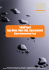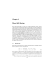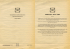# the nonlinear dynamics of a vibrating system modelled by a inverted

## Transcription

the nonlinear dynamics of a vibrating system modelled by a inverted
```THE NONLINEAR DYNAMICS OF A VIBRATING SYSTEM MODELLED BY A INVERTED
PENDULUM, WITH AN ELECTRODYNAMIC SHAKER
Ana Rosa Klinke1 , José Manoel Balthazar2 , Jorge Luiz Palacios Felix3 , Fábio Roberto Chavarette4
1,2
UNESP: Univ Estadual Paulista, Department of Statistics Applied Mathematics and Computation, PO Box 178, 13500-230, Rio Claro SP, Brazil, [email protected], [email protected]
3
Regional University of Northwestern of Rio Grande do Sul - Department of Physics Mathematical and Statistics, São Geraldo, Brazil,
[email protected]
4
UNESP: Univ Estadual Paulista, Department of Mathematics, Ilha Soltlteira, Brazil, [email protected]
Abstract: The technology of macro-electro-mechanical
systems has many applications on Engineering Sciences.
However, the design of such mechanical systems can be quite
challenging due to nonlinear effects, which may strongly affected the dynamics behavior. The physical model studied
is a macro-electro-mechanical coupling with an electrodynamic shaker, by using numerical simulations.
Keywords: Macro-Electro-Mechanical Systems, Nonlinear
Dynamics, Chaos.
1. INTRODUCTION
Micro-electro-mechanical systems (MEMS) are small
integrated devices or systems those combine electrical
and mechanical components. Examples of MEMS devices applications are emerging as the existing technology is applied to the miniaturization and integration of
conventional devices. Examples of MEMS devices applications,include inertial sensors, as an example. They
are used as an example in airbag-deployment sensors,
in automobiles, and as tilt or shock sensors Some details of (MEMS), may be easly find on the web address (http://en.wikipedia.org/wiki/Microelectromechanical
systems). However, the design of such dynamical systems,
may be quite challenging, due to their nonlinear effects,
which may strongly affect their dynamics behavior.
It is known that nonlinear systems, have been shown to
exhibit chaotic behavior, as well a periodic oscillations. A
chaotic system is a nonlinear deterministic system, which it
is very sensitive to small perturbations on its initial conditions, and its long time behavior it is unpredictable . It is well
known that the classical tuning-fork (MEMS) gyroscope,
contains a pair of masses that are driven to oscillate with
equal amplitude but in opposite directions. When rotated,
the Coriolis force creates an orthogonal vibration that can
be sensed by a variety of mechanisms. The Draper Lab gyroscope shown in Figure 1, uses a comb-type structures to drive
the tuning fork into resonance. Rotation causes the proof
masses to vibrate out of plane, and this motion is sensed capacitively with a custom CMOS ASIC. The micro-tuningfork gyroscope,presented in Figure 1, may be mathematically modeled(by a Macro Model)by being two in- verted
pendulums, which their motions, opposing set in support of
mass vertical and horizontal movement [1, 2]. We remarked
that and, include, the effects of the basis of excitation of the
pendulum (parametric excitation), used an electro-dynamics
shaker [3, 4]. Its nonlinear dynamical behavior was studied by [3, 4] basead on a Macro model. In this paper, we
consider two Macro models. They also presented rich nonlinear dynamical behavior . Next we will discuss new and
interesting models, separately.
Figure 1 – A tuning fork gyroscope with comb drive for commercial applications by Draper Lab
Proceedings of the 9th Brazilian Conference on Dynamics Control and their Applications
Serra Negra, SP - ISSN 2178-3667
1179
2. MATHEMATICAL MODEL I
are obtained
The simplified Macro-Mechanical-Mathematical Model
I, considered, consists of one inverted pendulum fixed in suspension mass, showed in Figure 2. The pendulum has a mass
m connected by a rod of length l. And are θ the angle of pendulum and stabilized by torsion springs, with strongly nonlinear, kθ = k + hθ2 . The suspension with mass M , and
spring constant Kx for X direction . Then the variables
of the problem are the displacement and the suspension of
mass in the horizontal respectively (two degree of Freedom).
1
(f1 + η1 f2 cos θ)
∆
(7)
1
(F1 f1 cos θ + f2 + F )
∆
(8)
θ̈ =
Ẍ =
where
∆ = 1 − F1 η1 cos θ2
f1 = −δ1 θ − λ1 θ3 − µ1 θ̇ + η1 g1 sin θ
f2 = −µ4 Ẋ − ωx 2 X − F1 θ̇2 sin θ
F = A cos Ωτ
(9)
(10)
(11)
(12)
Making x1 = θ, x2 = θ̇, x3 = X, x4 = Ẋ, has a system
of first order in the state variables
x˙1 = x2
x˙2 =
1
x˙4 = (F1 f1 cos x1 + f2 + F )
∆
Figure 2 – Simple model of the Macro-Electro-Mechanical
Model I
The governing equations of motion, for the MacroElectro-Mechanical model, defined by Figure 2, are
ml2 θ̈ + cl2 θ̇ + k1 θ +
hθ3 = mlẍ cos θ + ml(ÿ + g) sin θ
(1)
Mt ẍ + cx ẋ + kx x − ml(θ̈ cos θ − θ̇2 sin θ) = 0
(2)
Where Mt = M + m and g is the acceleration of gravity. They may be transformed into dimensionless equations,
making the following changes of variables:
kx
1
X = lxc , τ = ωe t, ωe = √LC
, ωx = M
ωe 2 ,
t
0
k1
h1
1 l1
F1 = m
M t lc , δ 1 = m 1 l1 2 ω e 2 , λ 1 = m 1 l 1 2 ω e 2 ,
µ1 = m1 lc112 ωe , η1 = ll1c , g1 = ωeg2 lc , µ4 = Mctxωe .
Considering the simplified motion in the horizontal direction and a excitation force on Y direction F = A cos Ωτ , the
equation of motion of the simplified system is given by:
θ̈ + δ1 θ + λ1 θ3 + µ1 θ̇ − η1 (Ẍ cos θ + g1 sin θ) = 0
2
2
Ẍ + µ4 Ẋ + ωx X − F1 (θ̈ cos θ − θ̇ sin θ) = F
(3)
(4)
Isolating θ̈ and Ẍ:
θ̈ − η1 Ẍ cos θ = −δ1 θ − λ1 θ3 − µ1 θ̇ + η1 g1 sin θ ≡ f1 (5)
Ẍ − F1 θ̈ cos θ = F − µ4 Ẋ − ωx 2 X − F1 θ̇2 ≡ f2 (6)
1
(f1 + η1 f2 cos x1 )
∆
x˙3 = x4
(13)
(14)
(15)
(16)
where
∆ = 1 − F1 η1 cos x1 2
f1 = −δ1 x1 − λ1 x1 3 − µ1 x2 + η1 g1 sin x1
f2 = −µ4 x4 − ωx 2 x3 − F1 x˙2 2 sin x1
F = A cos Ωτ
(17)
(18)
(19)
(20)
2.1. NUMERICAL SIMULATION RESULTS
The numerical simulations, were carried out, by using Matlabő, taken as the numerical integrator the RungeKutta method of fourth order algorithm with variable time
steplength. The following is presented an analysis of the behavior of each component of the proposed models, making
an approach on the nonlinear behavior.
The initial conditions of (θ, θ̇, X, Ẋ), were fixed as
[0.12, 0.01, 0.0025, 0.01].The equations parameters for the
oscillator are shown in Table 1 [1, 2, 5], are:
The numerical results of the equations 13, 14, 15, 16 is
illustrated through Figures 3, 4, 5.
The Figure 3(a) shows the phase portrait of (θ, θ̇) and Figure 3(b) shows the phase motion of (X, Ẋ). The time history
of angular displacement of θ in the time range 0 ≤ t ≤ 4000
is showed in Figure 4(a) and the time history of X is showed
by Figure 4(b)
Proceedings of the 9th Brazilian Conference on Dynamics Control and their Applications
Serra Negra, SP - ISSN 2178-3667
1180
Table 1 – Parameters for the chaotic oscillator.
Parameters Values
A
0.2
Ω
1.4
F1
0.1
µ4
0
µ1
0.01
ωx
1.0
η1
0.9
λ1
0.75
g1
1.0
δ1
1.0
2.2. PARAMETERS ANALYSIS
The following results, were obtained in order to observe
the effect of parameter bifurcation in equations 13, 14, 15,
16. The bifurcation parameter, change in the invervalo 0 ≤
P arameter ≤ 6 and other parameters of the equations, were
held constant.
Figure 6 shows the bifurcation diagram of the parameter µ4 , with the purpose of observing the damping effects
of the suspension mass on the oscillation of the pendulums
(X-direction). The analysis of results shows that when the
parameter µ4 is very close to 0, causing an instability in the
considered system.
Figure 3: (a) Phase Portrait of θ, (b) Phase Portrait of X.
Figure 6: Bifurcation Diagram of parameter µ4
Figure 7 shows the bifurcation diagram of the parameter
ωx , this parameter represents the natural excitement of the
system in the direction X. When this parameter adquive a
value between 1.2 and 1.4 the system becomes unstable.
Figure 4: (a) Time History of θ, (b)Time History of X.
Figure 5(a) illustrates the frequency spectrum of angular
displacement θ and Figure 5(b) the frequency spectrum of
motion X. According to the angular movement of the pendulum and the movement in the X direction ,we can see a
nonlinear interaction between the inverted pendulum and the
force excitation, evidenced by the three peaks, occurred in
the frequency spectrum.
Figure 7: Bifurcation Diagram of parameter ωx
Figure 5: (a)Frequency Spectrum of θ, (b) Frequency Spectrum of X.
Next,we present the results of the numerical simulations,
those were carried out, is done to observe the influence of the
parameter, λ1 , of the nonlinearity of stiffness of the pendulum. The bifurcation diagram showed the interaction of this
parameter, with the system illustrated in Figure 8. As ex-
Proceedings of the 9th Brazilian Conference on Dynamics Control and their Applications
Serra Negra, SP - ISSN 2178-3667
1181
pected, with increasing of the cubic nonlinearity coefficient
λ1 , the degree of instability of the system increases considerably. Increasing of instability is caused by the fact that this
parameter is a nonlinear coefficient of the system.
Figure 10: Bifurcation Diagram of parameter A
3. SIMPLIFIED MATHEMATICAL MODEL II
Figure 8: Bifurcation Diagram of parameter λ1
The bifurcation diagram of the parameter w, shown in
Figure 9, illustrates the effect of parameter oscillation frequency of the excitation force, F = A cos Ωτ , in the system.
We can observe the occurrence of instabilidade of the system,
when the parameter ranging from 0.4 to 1.3.
Figure 11 – Macro-Electro-Mechanical Model II, coupling with
an electrodynamic shaker
Figure 9: Bifurcation Diagram of parameter Ω
The study of the amplitude A shows the intensity of the
excitation force F = A cos Ωτ . The bifurcation diagram
of Figure 10 shows the interaction between this parameter
and the system. It is possible to observe that with increasing value of A, there is an instability occurring in the system, which increasing along with increasing A. This result
is in complete agreement with the proposed model, because
of higher the level of excitement of the pendulum and the
suspension of mass those suffering the greater the instability
generated in the system.
The Macro-Mechanical-Mathematical Model II, considered here, was based on initial experimental work on the basis of excitation of a pendulum (parametric excitation) by using electrodynamic shaker made by [3, 4]. The mechanism
is studied here, was implemented as excited at its base by
an electrodynamic shaker as shown in Figure 11.The electrodynamic shaker acts on the suspension of mass M. The coupling between both parties is achieved by the electromagnetic
force due to the permanent magnet Fem = K q̇ (Fem = lc B,
where lc e B are the length of the conductor and the magnetic
field, respectively). This generates a force of Laplace on the
mechanics and the Lenz electromotive voltage in the power
[1, 2, 5]. The electrical system consists of resistor R, an inductor L, a capacitor C and a source of sinusoidal voltage
e(t) = e0 cos Ωt(e0 and Ω are the amplitude and frequency
respectively; t is the time), all connected in series. In this
model, the voltage of the capacitor is a nonlinear function of
instantaneous electric charge q, defined as:
Proceedings of the 9th Brazilian Conference on Dynamics Control and their Applications
Serra Negra, SP - ISSN 2178-3667
1182
Vc =
1
q + α3 q 3
C0
(21)
θ̈ − η1 (Ẍ cos θ − Ÿ sin θ) =
−δ1 θ − λ1 θ3 − µ1 θ̇ + η1 g1 sin θ ≡ f1
where C0 is the linear value of C and α3 is the nonlinear
coefficient depending on the type of capacitor to be used [1,
2, 5].
The kinetic energy and potential energy of the system are
given by
Ẍ − F1 θ̈ cos θ =
−µ4 Ẋ − ωx 2 X − F1 θ̇2 sin θ ≡ f2
Ÿ − F1 θ̈ sin θ =
−µ5 Ẏ − ωy 2 Y + F1 θ̇2 cos θ + γ1 Q̇ ≡ f3
3
1
1
T = Mt ẋ2 + Mt ẏ 2 −
2
2
1 2 2 1 2
ml(ẋθ̇ cos θ + ẏ θ̇ sin θ) + ml θ̇ + Lq̇
2
2
ky 2 kx 2
V = Mt gy + mg(l cos θ + y) + y + x +
2
2
1 2 1 2
1 2 α3 4
q
K q̇y + kθ + hθ +
q +
2
4
2C0
4
Q̈ = −µ3 Q̇ − Q − λ3 Q − γ2 Ẏ + E0 cos (ωτ )
(22)
2
1
1
1
1
c(lθ̇) + Rq̇ 2 + cx ẋ2 + cy ẏ 2
2
2
2
2
x˙1 = x2
1
x˙2 = (f1 + η1 f2 cos x1 + η1 f3 sin x1 )
∆
x˙3 = x4
1
x˙4 = (F1 f1 cos x1 + f2 )
∆
x˙5 = x6
1
x˙6 = (F1 f1 sin x1 + f3 )
∆
x˙7 = x8
(23)
(24)
The motion equation of the system is given by:
2
2
ml θ̈ + cl θ̇ + k1 θ +
hθ3 = mlẍ cos θ + ml(ÿ + g) sin θ (25)
Mt ẍ + cx ẋ + kx x − ml(θ̈ cos θ − θ̇2 sin θ) = 0 (26)
Mt ÿ + cy ẏ + ky y − ml(θ̈ sin θ + θ̇2 cos θ) − K q̇ = 0 (27)
1
Lq̈ + Rq̇ +
q + α3 q 3 + K ẏ = E0 cos (Ωt) (28)
C0
Where Mt = M + m and g is the acceleration of gravity.
Making the following changes of variables:
X=
x
lc ,
Y =
y
lc ,
Q=
√ 1 , ωx =
LC0
1
F1 = Mml
, δ1 = mlk2 1ωe 2 , λ1 = mlh2 ω
2,
t lc
e
2
α3 q0
c1
lc
λ3 = Lωe 2 , µ1 = ml2 ωe , η1 = l , g1 = ωeg2 lc ,
c
R
, µ5 = Mtyωe ,
µ4 = Mctxωe , µ3 = Lω
e
Klc
0
γ1 = MKq
, γ2 = Lω
, E0 = Lωee02 q0 , ω = ωΩe .
t ω e lc
e q0
ωe =
3
x˙8 = −µ3 x8 − x7 − λ3 x7 − γ2 x6 + E0 cos (ωτ )
(35)
(36)
(37)
(38)
(39)
(40)
(41)
(42)
(43)
(44)
where
∆ = 1 − F1 η1 cos x1 2 − F1 η1 sin x1 2
f1 = −δ1 x1 − λ1 x1 3 − µ1 x2 + η1 g1 sin x1
f2 = −µ4 x4 − ωx 2 x3 − F1 x2 2 sin x1
2
2
f3 = −µ5 x4 − ωy x5 + F1 x2 cos x1 + γ1 x8
q
q0 ,
τ = ωe t,
ky
kx
,
ω
=
2
y
Mt ω e
Mt ω e 2 ,
(34)
Making x1 = θ, x2 = θ̇, x3 = X, x4 = Ẋ, x5 = Y ,
x6 = Ẏ , x7 = Q, x8 = Q̇, we will obtain the system of first
order in the state variables
The dissipation energy function of Rayleigh’s is given by
D=
(33)
(45)
(46)
(47)
(48)
(49)
3.1. NUMERICAL SIMULATION RESULTS
The initial conditions of (θ, θ̇, X, Ẋ, Y, Ẏ , Q, Q̇) fixed
as being (0.12, 0.01, 0.025, 0.01, 0.1, 0.01, 0.3, 0.01) respectively. The equations parameters for the oscillator, which was
fabricated using standard silicon on insulator processing device shown in Table 1 [1, 2, 5], are:
we will obtain
θ̈ + δ1 θ + λ1 θ3 +
µ1 θ̇ − η1 (Ẍ cos θ + (Ÿ + g1 ) sin θ) = 0
Ẍ + µ4 Ẋ + ωx 2 X −
Table 2 – Parameters for the chaotic oscillator, coupling with an
electrodynamic shaker.
(29)
F1 (θ̈ cos θ − θ̇2 sin θ) = 0
(30)
F1 (θ̈ sin θ + θ̇2 cos θ) + γ1 Q̇ = 0
Q̈ + µ3 Q̇ + Q + λ3 Q3 + γ2 Ẏ = E0 cos (ωτ )
(31)
(32)
2
Ÿ + µ5 Ẏ + ωy Y −
Isolating θ̈, Ẍ, Ÿ and Q̈:
Parameters Values Parameters Values
F1
0.1
µ4 , µ5
0
µ1
0.01
µ3
0.05
ωx
1.0
ωy
1.0
η1
0.9
γ2
0.2
λ1
0.75
ω
1.5
g1
1.0
ωe
1.0
δ1
1.0
E0
0.24
λ3
1.0
γ1
0.4
Proceedings of the 9th Brazilian Conference on Dynamics Control and their Applications
Serra Negra, SP - ISSN 2178-3667
1183
The numerical results of the equations 37, 38, 39, 40, 41,
42, 43, 44 are illustrated by Figures 12 to 19. These results
are all givwen is in the time range 0 ≤ t ≤ 5000.
The Figure 12(a) shows the phase portrait (θ, θ̇) and Figure 12(b) shows the frequency spectrum of θ. The time history of angular displacement of θ̇ is shows by Figure 13(a)
and the time history of θ is shows by Figure 13(b). In this
system, when we consider the action of the electrodynamic
shaker, we obtain that the angular movement of the inverted
pendulum is stabilized. This is evidenced by the frequency
spectrum , which show only one peak.
Figure 15: (a)Time History of Ẋ, (b)Time History of X.
In the Figure 16(a) and 16(b) shows the phase portait
(Y, Ẏ ) and the frequency spectrum of Y , respectively. The
Figure 17(a) and 17(b) illustrates the time history of Ẏ and
the time history of Y , respectively. The Y movement has a
nonlinear behavior (shown by the frequency spectrum containing multiple peaks).
Figure 12: (a)Phase portrait, (b)Frequency Spectrum
Figure 16: (a)Phase Portrait of Y , (b)Frequency Spectrum of Y .
Figure 13: (a)Time History of θ̇, (b)Time History of θ.
The Figure 14(a) shows the phase portrait (X, Ẋ) and
Figure 14(b) shows the frequency spectrum of X. The time
history of Ẋ is shows by Figure 15(a) and the time history of
X is shows by Figure 15(b). In the motion X has a periodic
behavior, as illustrated by the phase portrait.
Figure 17: (a)Time History of Ẏ , (b)Time History of Y .
The behavior of the electrodynamic shaker it is illustrated
in the phase portrait (Q, Q̇), Figure 18(a). The Figure 18(b)
shows the frequency spectrum of Q. The Figure 19(a) and
19(b) illustrates the time history of Q̇ and the time history of
Q, respectively.
Figure 14: (a)Phase Portrait of θ, (b)Frequency Spectrum of X.
Figure 18: (a)Phase Portrait of Q, (b)Frequency Spectrum of Q.
Proceedings of the 9th Brazilian Conference on Dynamics Control and their Applications
Serra Negra, SP - ISSN 2178-3667
1184
Figure 19: (a)Time History of Q̇, (b)Time History of Q.
3.2. PARAMETERS ANALYSIS
The next results of numerical simulations, were obtained,
in order to observe the effect of parameter bifurcation in this
equations 37, 38, 39, 40, 41, 42, 43, 44. The bifurcation
parameter change in the time range 0 ≤ P arameter ≤ 5
and other parameters of the equations were held constant.
Figure 20 shows the bifurcation diagram of the parameter µ4 , with the purpose of observing the damping effects of
the suspension mass on the oscillation of the pendulums (Xdirection) and the interaction with the eletrodynamic shaker.
The analysis of results shows that when the parameter µ4
is very close to 0 causes an instability in the system. This
result resembles the results were obtained with the MacroElectro-Mechanical Model I. We conclude that the damping
effect on both systems cause instability to values close to 0,
this is because the damping effect of the oscillation decreases
in directions X and Y .
Figure 21: Bifurcation Diagram of parameter ωx
Figure 22 shows the bifurcation diagram of the parameter
ωy , which represents the natural excitement of the system in
the Y direction, analyzing the results we have obtained that
the system becomes unstable for values of ωy greater than 1.
Figure 22: Bifurcation Diagram of parameter ωy
Figure 20: Bifurcation Diagram of parameter µ4
The parameter λ1 represents the nonlinear coefficient of
the pendulum, and the bifurcation diagram shows the interaction of this parameter, the system is illustrated in Figure 23.
Analyzing the results, we noted that the system behaves unstable, when the coefficient of cubic nonlinearity reached values above 4.5.
Figure 21 shows the bifurcation diagram of the parameter
ωx , which represents the natural excitement of the system in
the X direction. The system becomes unstable in the range
of values from 0 to 2.
Proceedings of the 9th Brazilian Conference on Dynamics Control and their Applications
Serra Negra, SP - ISSN 2178-3667
1185
chanical Systems and Signal Processing, pp. 1146–1156,
2008.
 X. Xu, E. Pavlovskaia, M. wiercigroch, F. Romeo,
and S. Lenci., “Dynamic interactions between parametric pendulum and electo-dynamical shaker,” ZAMM Z.
Angew. Math. Mech., pp. 172–186, 2007.
 J. M. Balthazar, J. L. P. Felix, and M. L. R. F. Reyolando,
“On an energy transfer and nonlinear, nonideal and
chaotic behavior of a macro tuning fork beam(tfb), under an electro-dynamical shaker excitation(eds).,” Proceedings of 11th Pan-American Congress of Applied Mechanics, 2010.
Figure 23: Bifurcation Diagram of parameter λ1
 J. L. P. Felix and J. M. Balthazar, “Comments on a nonlinear and non-ideal electromechanical damping vibration absorber. sommerfeld effect and energy transfer,”
Nonlinear Dynamics, pp. 1–11, 2009.
4. CONCLUSION
In this paper, the study of an macro-electro-mechanical,
firstly modeled by one inverted pendulum fixed in a suspension mass (Macro-Mechanical-Mathematical Model I), was
done by numerical simulations. With the analysis of results
obtained in 2.1 Session, we obtained a nonlinear interaction
between the inverted pendulum and the force excitation, evidenced by the three peaks, occurred in the frequency spectrum, Figure 5. The results of the bifurcation diagrams, Session 2.2, showed that the parameters λ1 , A, Ω, ωx generated
instability in the system, as illustrated by Figures 8, 9, 7, 10,
respectively.
The second system, macro-electro-mechanical (MacroMechanical-Mathematical Model II), showed in the results
obtained with numerical simulations that the interaction of
electrodynamic shaker with the pendulum has generated a
stability in the angular motion of the pendulum, as illustrated
by Figure 12, 13. The behavior of electrodynamic shaker is
periodic motion, as illustrated by Figure 18, 19. The analysis of nonlinear effects are of great importance for macroelectro-mechanical systems, because they can strongly affect
the dynamics. We found the interdependence of inverted pendulums and eletrodynamic shaker, and provide evidence of
the influence of motion of the pendulum about the vertical
excitation provided by eletrodynamic shaker. Through this
interaction was detected the presence of nonlinear phenomena illustrated through Figure 14(b).
ACKNOWLEDGMENTS
The authors acknowledge the financial support by
FAPESP.
REFERENCES
 Y. Lee and Z. C. Feng, “Feasibility study of parametric
excitations of a tuning fork microgyroscope,” Proceedings of IMECE, 2004.
 Y. Lee, P. F. Pai, and Z. C. Feng, “Nonlinear complex
response of a parametrically excited tuning fork,” Me-
Proceedings of the 9th Brazilian Conference on Dynamics Control and their Applications
Serra Negra, SP - ISSN 2178-3667
1186
```

### Elektrostatika: Hukum Coulomb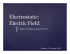### wdgl eagle 98.1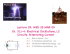### Raising the Bar on Beauty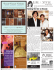### Lorenz system II - Harvard Mathematics Department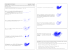### On Foucault`s Pendulum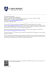### Métodos multipaso coseno para ecuaciones diferenciales### antiderivative of lnx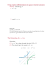### Document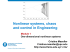### Modélisation et simulation des turbines éoliennes en vue du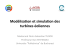### The Divergence Theorem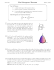### Resolvers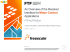### Squirmers with swirl: a model for Volvox swimming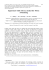### to see the Cosmetology PowerPoint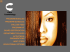### Trigonometric functions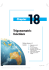### Active Control of Separated Flow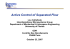### SHAKER DOOR COLLECTION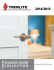### A family of newforms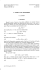### Introduction to Differential Equations### vn ncom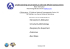### SkidProof Any floor. Non